GFG App
Open AppBrowser
Continue

# Binary Number System – Definition, Conversion, Examples

A binary number system is one of the four types of number systems, and it is used to define a number in a binary system. A binary number system represents a number in terms of only two digits, i.e., 0 (zero) and 1 (one). In the word “binary”, “bi” means “two”. As a result, this draws the line back to the representation of a number using the numbers 0 and 1 only. The base-2 numeral system is used to represent binary numbers. For example, (1101)2 is a binary number where 2 is the radix. Each digit in the binary number system is said to be a “bit”.

This number system is widely used in computers. All inputs given to a computer are decoded by it into a series of 0’s or 1’s before being processed further since a computer can only understand binary information, which is represented by the numbers 0 or 1. It is simple to convert a decimal number into a binary number and vice-versa. The notations for decimal numbers and binary numbers are different. For example, a decimal is represented as (15)10 where 10 is the base of the decimal number, and the corresponding binary number is represented as (1111)2 where 2 is the base of a binary number.

### Binary to Decimal Conversion

A binary number is converted into a decimal number by multiplying each digit of the binary number by the power of either 1 or 0 to the corresponding power of 2. Let us consider that a binary number has n digits, B = an-1…a3a2a1a0. Now, the corresponding decimal number is given as D= (an-1 × 2n-1) +…+(a3 × 23) + (a2 × 22) + (a1 × 21) + (a0 × 20).

Let us go through an example to understand the concept better.

Example: Convert (10011)2 to a decimal number.

Solution:

The given binary number is (10011)2.

(10011)2 = (1 × 24) + (0 × 23) + (0 × 22) + (1 × 21) + (1 × 20)

= 16 + 0 + 0 + 2 + 1 = (19)10

Hence, the binary number (10011)2 is expressed as (19)10.

### Decimal to Binary Conversion

A decimal number is converted into a binary number by dividing the given decimal number by 2 continuously until we get the quotient as 1, and we write the numbers from downwards to upwards.

Let us go through an example to understand the concept better.

Example: Convert (28)10 into a binary number.

Solution: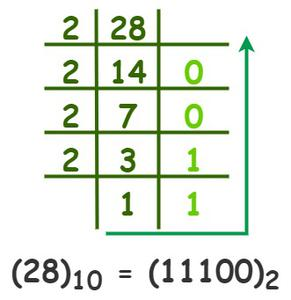Hence, (28)10 is expressed as (11100)2.

### Arithmetic operation on Binary numbers

The result of the addition of two binary numbers is also a binary number. To obtain the result of the addition of two binary numbers, we have to add the digit of the binary numbers by digit. Remember the table given below while adding two binary numbers.

Binary Subtraction

The result of the subtraction of two binary numbers is also a binary number. To obtain the result of the subtraction of two binary numbers, we have to subtract the digit of the binary numbers by digit. Remember the table given below while subtracting two binary numbers.

Binary Multiplication

The multiplication process of binary numbers is similar to the multiplication of decimal numbers. The rules for multiplying any two binary numbers are given as follows:

Binary Division

The division method for binary numbers is similar to that of the decimal number division method.

Let us go through an example to understand the concept better.

Example: Divide (101101)2 by (110)2.

Solution: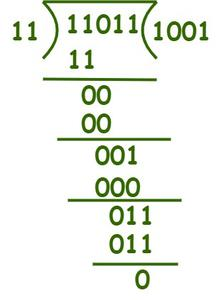#### 1’s and 2’s complement of a Binary Number

• 1’s complement of a binary number is obtained by inverting the digits of the binary number.

Example: Determine the 1’s complement of (10011)2.

Solution:

The given binary number is (10011)2.

Now, to find its 1’s complement, we have to invert the digits of the given number.

Thus, 1’s complement of (10011)2 is (01100)2.

• 2’s complement of a binary number is obtained by inverting the digits of the binary number and then by adding 1 to the least significant bit.

Example: Determine the 2’s complement of (1011)2.

Solution:

The given binary number is (1011)2.

To find the 2’s complement, first find its 1’s complement, i.e., (0100)2.

Now, by adding 1 to the least significant bit, we get (0101)2.

Hence, the 2’s complement of (1011)2 is (0101)2.

### Problems based on Binary Number System

Problem 1: Convert the decimal number (98)10 into binary.

Solution:

To obtain the binary number for 98, we have to divide it continuously by 2.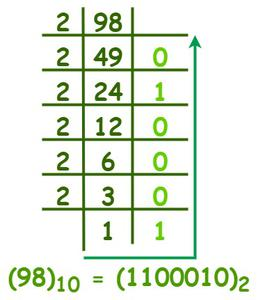Thus, the binary number for (98)10 is (1100010)2.

Problem 2: Convert the binary number (1010101)2 to a decimal number.

Solution:

The given binary number is (1011101)2

= (1 × 20) + (0 × 21) + (1 × 22) + (0 × 23) + (1 × 24) + (0 × 25) + (1 ×26)

= 1 + 0 + 4 + 0 + 16 + 0 + 64

= (85)10

Thus, the binary number 1010101 is 85 in decimal.

Problem 3: Divide (11110)2 by (101)2.

Solution: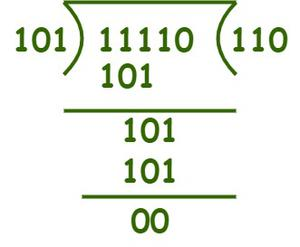Problem 4: Add (11011)2 and (10100)2.

Solution: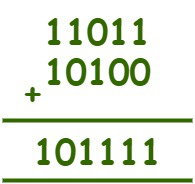Hence, (11011)2 + (10100)2 =  (101111)2.

Problem 5: Subtract (11010)2 and (10110)2.

Solution: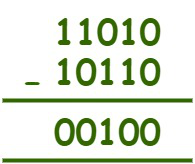Hence, (11010)2 – (10110)2 = (00100)2.

Problem 6: Multiply (1110)2 and (1001)2.

Solution: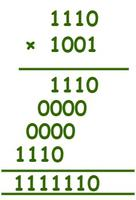Thus, (1110)2 × (1001)2 = (1111110)2.

My Personal Notes arrow_drop_up